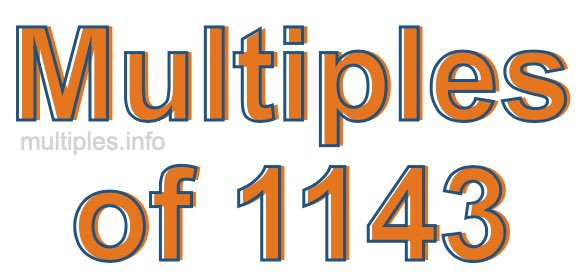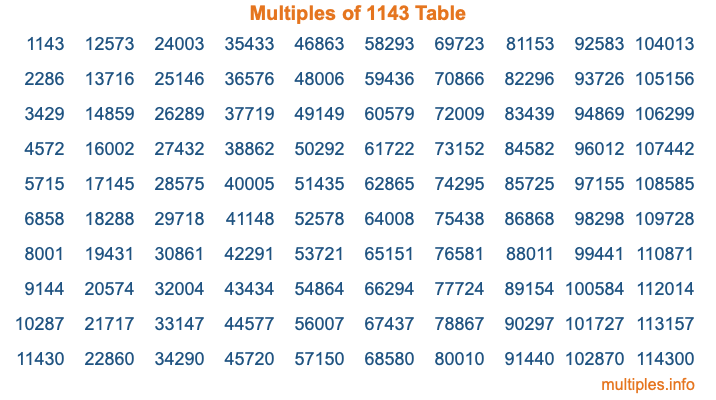Multiples of 1143Welcome to the Multiples of 1143 page. Here we will first teach you everything you will ever need to know about the multiples of 1143, and then give you a study guide summary of everything we taught you to make sure you remember it all. Use this page to look up facts and learn information about the multiples of 1143. This page will make you a multiples of one thousand one hundred forty-three expert!

Definition of Multiples of 1143
Multiples of 1143 are all the numbers that when divided by 1143 equal an integer. Each of the multiples of 1143 are called a multiple. A multiple of 1143 is created by multiplying 1143 by an integer.

Therefore, to create a list of multiples of 1143, you start with 1 multiplied by 1143, then 2 multiplied by 1143, then 3 multiplied by 1143, and so on for as long as you want. Thus, the list of the first five multiples of 1143 is 1143, 2286, 3429, 4572, and 5715. To see a larger list of multiples of 1143, see the printable image of Multiples of 1143 further down on this page. We also have a category where you can choose any nth multiple of 1143.

Multiples of 1143 Checker
The Multiples of 1143 Checker below checks to see if any number of your choice is a multiple of 1143. In other words, it checks to see if there is any number (integer) that when multiplied by 1143 will equal your number. To do that, we divide your number by 1143. If the the quotient is an integer, then your number is a multiple of 1143.

Is  a multiple of 1143?

Least Common Multiple of 1143 and ...
A Least Common Multiple (LCM) is the lowest multiple that two or more numbers have in common. This is also called the smallest common multiple or lowest common multiple and is useful to know when you are adding our subtracting fractions. Enter one or more numbers below (1143 is already entered) to find the LCM.

Check out our LCM Calculator if you need more details about the Least Common Multiple or if you need the LCM for different numbers for adding and subtraction fractions.

nth Multiple of 1143
As we stated above, 1143 is the first multiple of 1143, 2286 is the second multiple of 1143, 3429 is the third multiple of 1143, and so on. Enter a number below to find the nth multiple of 1143.

th multiple of 1143

Multiples of 1143 vs Factors of 1143
1143 is a multiple of 1143 and a factor of 1143, but that is where the similarities end. All postive multiples of 1143 are 1143 or greater than 1143. All positive factors of 1143 are 1143 or less than 1143.

Below is the beginning list of multiples of 1143 and the factors of 1143 so you can compare:

Multiples of 1143: 1143, 2286, 3429, 4572, 5715, etc.

Factors of 1143: 1, 3, 9, 127, 381, 1143

As you can see, the multiples of 1143 are all the numbers that you can divide by 1143 to get a whole number. The factors of 1143, on the other hand, are all the whole numbers that you can multiply by another whole number to get 1143.

It's also interesting to note that if a number (x) is a factor of 1143, then 1143 will also be a multiple of that number (x).

Multiples of 1143 vs Divisors of 1143
The divisors of 1143 are all the integers that 1143 can be divided by evenly. Below is a list of the divisors of 1143.

Divisors of 1143: 1, 3, 9, 127, 381, 1143

The interesting thing to note here is that if you take any multiple of 1143 and divide it by a divisor of 1143, you will see that the quotient is an integer.

Multiples of 1143 Table
Below is an image of the first 100 multiples of 1143 in a table. The table is in chronological order, column by column. The first column has the first ten multiples of 1143, the second column has the next ten multiples of 1143, and so on.The Multiples of 1143 Table is also referred to as the 1143 Times Table or Times Table of 1143. You are welcome to print out our table for your studies.

Negative Multiples of 1143
Although not often discussed or needed in math, it is worth mentioning that you can make a list of negative multiples of 1143 by multiplying 1143 by -1, then by -2, then by -3, and so on, to get the following list of negative multiples of 1143:

-1143, -2286, -3429, -4572, -5715, etc.

Multiples of 1143 Summary
Below is a summary of important Multiples of 1143 facts that we have discussed on this page. To retain the knowledge on this page, we recommend that you read through the summary and explain to yourself or a study partner why they hold true.

There are an infinite number of multiples of 1143.

A multiple of 1143 divided by 1143 will equal a whole number.

1143 divided by a factor of 1143 equals a divisor of 1143.

The nth multiple of 1143 is n times 1143.

The largest factor of 1143 is equal to the first positive multiple of 1143.

1143 is a multiple of every factor of 1143.

1143 is a multiple of 1143.

A multiple of 1143 divided by a divisor of 1143 equals an integer.

1143 divided by a divisor of 1143 equals a factor of 1143.

Any integer times 1143 will equal a multiple of 1143.

Multiples of a Number
Here you can get the multiples of another number, all with the same attention to detail as we did for multiples of 1143 on this page.

Multiples of
Multiples of 1144
Did you find our page about multiples of one thousand one hundred forty-three educational? Do you want more knowledge? Check out the multiples of the next number on our list!